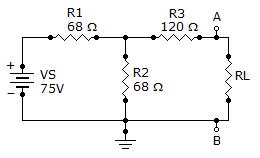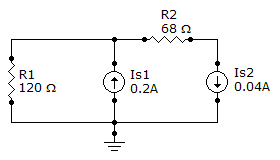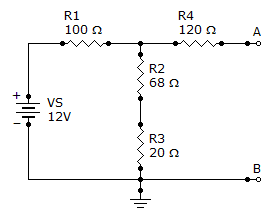# Electrical Engineering - Circuit Theorems and Conversions

Exercise : Circuit Theorems and Conversions - General Questions
21.
Find RN for the circuit given.3468120154Explanation:
No answer description is available. Let's discuss.

22.
Referring to circuit given, if R1 is changed to a 68resistor, what will be the current through it?0.16 A
0.24 A
0.2 A
0.04 A
Explanation:
No answer description is available. Let's discuss.

23.
Find the Thevenin equivalent (VTH and RTH) between terminals A and B of the circuit given.562 mV, 1675.62 V, 1675.62 V, 188562 mV, 188Explanation:
No answer description is available. Let's discuss.

24.
Referring to the given circuit, determine VTH and RTH if a 68resistor is connected in parallel across R2 and R3.3.3 V, 148330 mV, 1483.3 V, 68330 mV, 68A 12 mA current source has an internal resistance, RS, of 1.2 k. The equivalent voltage source is